## Occurrence, Preparation, and Properties of Transition Metals and Their Compounds

### Learning Objectives

By the end of this module, you will be able to:

• Outline the general approach for the isolation of transition metals from natural sources
• Describe typical physical and chemical properties of the transition metals
• Identify simple compound classes for transition metals and describe their chemical properties

Transition metals are defined as those elements that have (or readily form) partially filled d orbitals. As shown in Figure 1, the d-block elements in groups 3–11 are transition elements. The f-block elements, also called inner transition metals (the lanthanides and actinides) also meet this criterion, because the d orbital is partially occupied before the f orbitals. The d orbitals fill with the copper family (group 11); for this reason, the next family (group 12) are technically not transition elements. However, the group 12 elements do display some of the same chemical properties and are commonly included in discussions of transition metals. Some chemists do treat the group 12 elements as transition metals.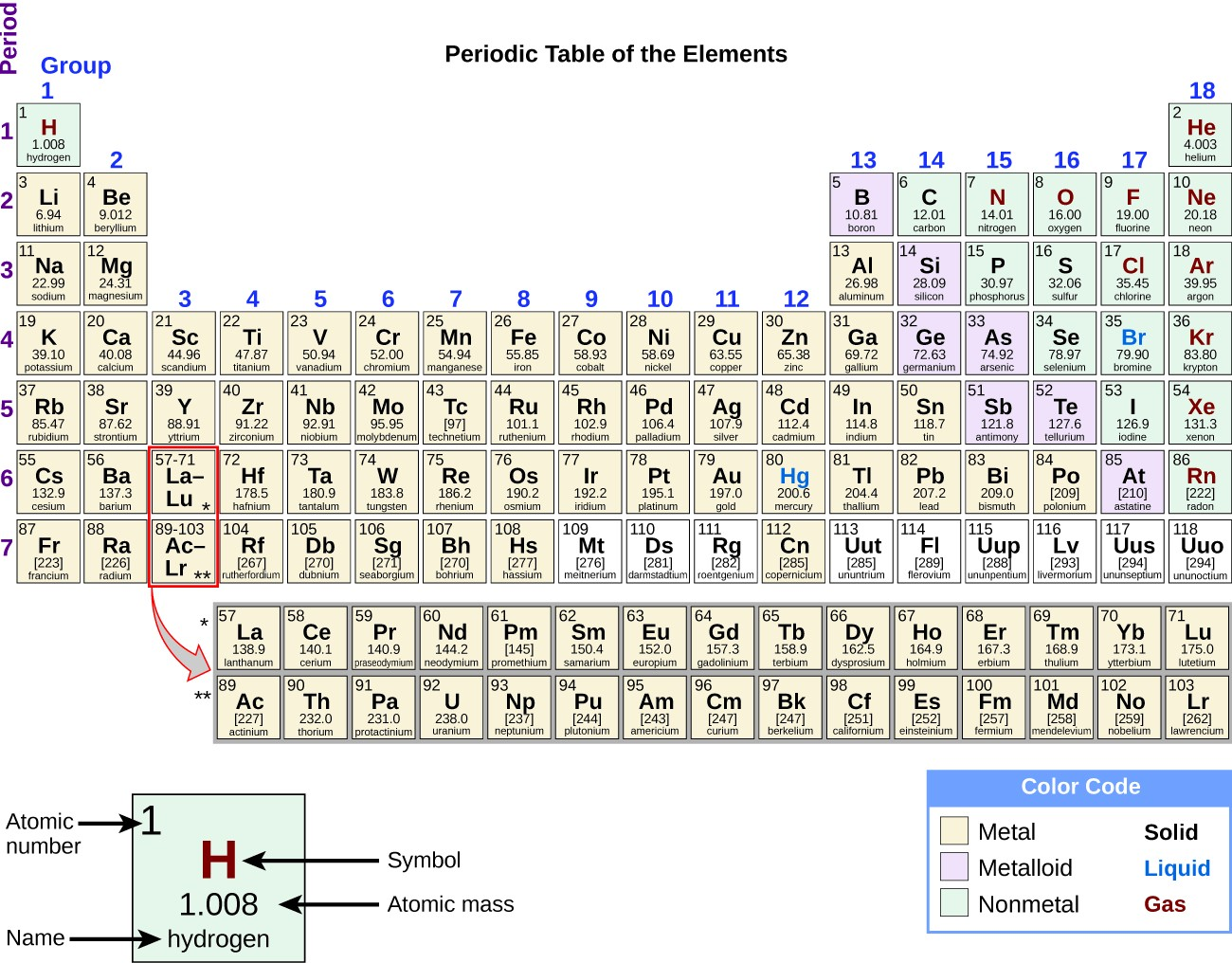Figure 1. The transition metals are located in groups 3–11 of the periodic table. The inner transition metals are in the two rows below the body of the table.

The d-block elements are divided into the first transition series (the elements Sc through Cu), the second transition series (the elements Y through Ag), and the third transition series (the element La and the elements Hf through Au). Actinium, Ac, is the first member of the fourth transition series, which also includes Rf through Rg.

The f-block elements are the elements Ce through Lu, which constitute the lanthanide series (or lanthanoid series), and the elements Th through Lr, which constitute the actinide series (or actinoid series). Because lanthanum behaves very much like the lanthanide elements, it is considered a lanthanide element, even though its electron configuration makes it the first member of the third transition series. Similarly, the behavior of actinium means it is part of the actinide series, although its electron configuration makes it the first member of the fourth transition series.

### Example 1: Valence Electrons in Transition Metals

Review how to write electron configurations, covered in the chapter on electronic structure and periodic properties of elements. Recall that for the transition and inner transition metals, it is necessary to remove the s electrons before the d or f electrons. Then, for each ion, give the electron configuration:

1. cerium(III)
3. Ti2+
4. Am3+
5. Pd2+

For the examples that are transition metals, determine to which series they belong.

Give an example of an ion from the first transition series with no d electrons.

### Uses of Lanthanides in Devices

Lanthanides (elements 57–71) are fairly abundant in the earth’s crust, despite their historic characterization as rare earth elements. Thulium, the rarest naturally occurring lanthanoid, is more common in the earth’s crust than silver (4.5 × 10-5% versus 0.79 × 10-5% by mass). There are 17 rare earth elements, consisting of the 15 lanthanoids plus scandium and yttrium. They are called rare because they were once difficult to extract economically, so it was rare to have a pure sample; due to similar chemical properties, it is difficult to separate any one lanthanide from the others. However, newer separation methods, such as ion exchange resins similar to those found in home water softeners, make the separation of these elements easier and more economical. Most ores that contain these elements have low concentrations of all the rare earth elements mixed together.

The commercial applications of lanthanides are growing rapidly. For example, europium is important in flat screen displays found in computer monitors, cell phones, and televisions. Neodymium is useful in laptop hard drives and in the processes that convert crude oil into gasoline. Holmium is found in dental and medical equipment. In addition, many alternative energy technologies rely heavily on lanthanoids. Neodymium and dysprosium are key components of hybrid vehicle engines and the magnets used in wind turbines.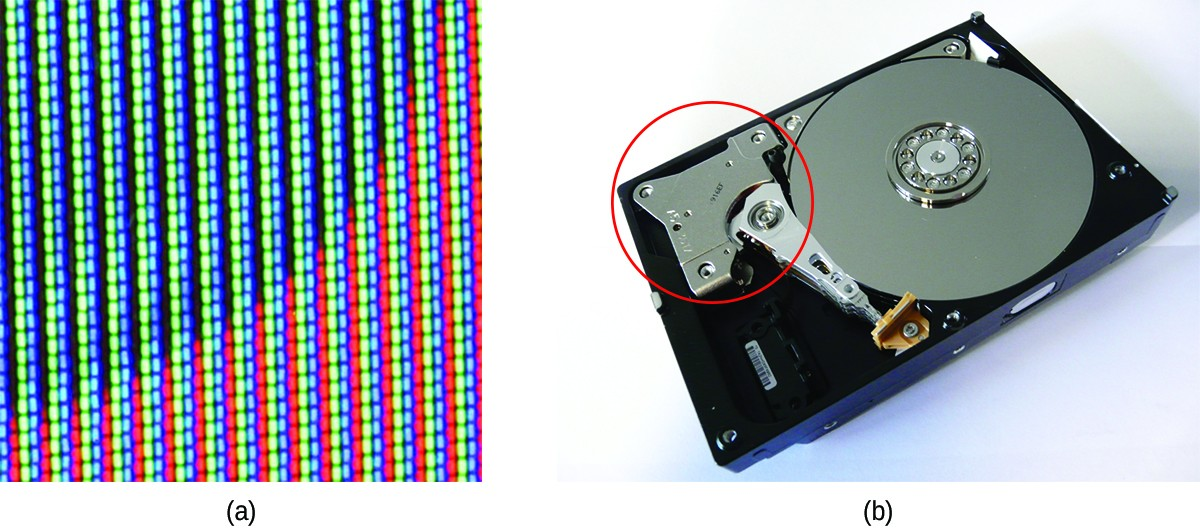Figure 2. (a) Europium is used in display screens for televisions, computer monitors, and cell phones. (b) Neodymium magnets are commonly found in computer hard drives. (credit b: “KUERT Datenrettung”/Flickr)

As the demand for lanthanide materials has increased faster than supply, prices have also increased. In 2008, dysprosium cost $110/kg; by 2014, the price had increased to$470/kg. Increasing the supply of lanthanoid elements is one of the most significant challenges facing the industries that rely on the optical and magnetic properties of these materials.

The transition elements have many properties in common with other metals. They are almost all hard, high-melting solids that conduct heat and electricity well. They readily form alloys and lose electrons to form stable cations. In addition, transition metals form a wide variety of stable coordination compounds, in which the central metal atom or ion acts as a Lewis acid and accepts one or more pairs of electrons. Many different molecules and ions can donate lone pairs to the metal center, serving as Lewis bases. In this chapter, we shall focus primarily on the chemical behavior of the elements of the first transition series.

## Properties of the Transition Elements

Transition metals demonstrate a wide range of chemical behaviors. As can be seen from their reduction potentials (see Ionization Constants of Weak Acids, some transition metals are strong reducing agents, whereas others have very low reactivity. For example, the lanthanides all form stable 3+ aqueous cations. The driving force for such oxidations is similar to that of alkaline earth metals such as Be or Mg, forming Be2+ and Mg2+. On the other hand, materials like platinum and gold have much higher reduction potentials. Their ability to resist oxidation makes them useful materials for constructing circuits and jewelry.

Ions of the lighter d-block elements, such as Cr3+, Fe3+, and Co2+, form colorful hydrated ions that are stable in water. However, ions in the period just below these (Mo3+, Ru3+, and Ir2+) are unstable and react readily with oxygen from the air. The majority of simple, water-stable ions formed by the heavier d-block elements are oxyanions such as ${\text{MoO}}_{4}{}^{2-}$ and ${\text{ReO}}_{4}{}^{-}.$

Ruthenium, osmium, rhodium, iridium, palladium, and platinum are the platinum metals. With difficulty, they form simple cations that are stable in water, and, unlike the earlier elements in the second and third transition series, they do not form stable oxyanions.

Both the d– and f-block elements react with nonmetals to form binary compounds; heating is often required. These elements react with halogens to form a variety of halides ranging in oxidation state from 1+ to 6+. On heating, oxygen reacts with all of the transition elements except palladium, platinum, silver, and gold. The oxides of these latter metals can be formed using other reactants, but they decompose upon heating. The f-block elements, the elements of group 3, and the elements of the first transition series except copper react with aqueous solutions of acids, forming hydrogen gas and solutions of the corresponding salts.

Transition metals can form compounds with a wide range of oxidation states. Some of the observed oxidation states of the elements of the first transition series are shown in Figure 3. As we move from left to right across the first transition series, we see that the number of common oxidation states increases at first to a maximum towards the middle of the table, then decreases. The values in the table are typical values; there are other known values, and it is possible to synthesize new additions. For example, in 2014, researchers were successful in synthesizing a new oxidation state of iridium (9+).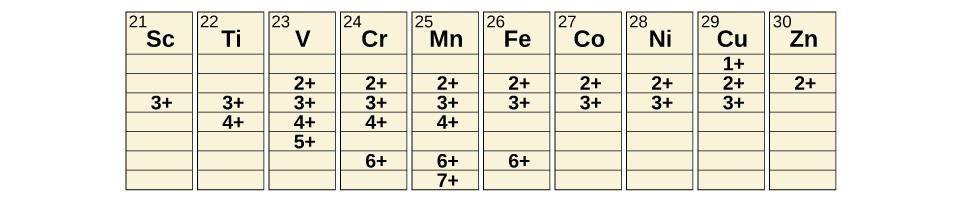Figure 3. Transition metals of the first transition series can form compounds with varying oxidation states.

For the elements scandium through manganese (the first half of the first transition series), the highest oxidation state corresponds to the loss of all of the electrons in both the s and d orbitals of their valence shells. The titanium(IV) ion, for example, is formed when the titanium atom loses its two 3d and two 4s electrons. These highest oxidation states are the most stable forms of scandium, titanium, and vanadium. However, it is not possible to continue to remove all of the valence electrons from metals as we continue through the series. Iron is known to form oxidation states from 2+ to 6+, with iron(II) and iron(III) being the most common. Most of the elements of the first transition series form ions with a charge of 2+ or 3+ that are stable in water, although those of the early members of the series can be readily oxidized by air.

The elements of the second and third transition series generally are more stable in higher oxidation states than are the elements of the first series. In general, the atomic radius increases down a group, which leads to the ions of the second and third series being larger than are those in the first series. Removing electrons from orbitals that are located farther from the nucleus is easier than removing electrons close to the nucleus. For example, molybdenum and tungsten, members of group 6, are limited mostly to an oxidation state of 6+ in aqueous solution. Chromium, the lightest member of the group, forms stable Cr3+ ions in water and, in the absence of air, less stable Cr2+ ions. The sulfide with the highest oxidation state for chromium is Cr2S3, which contains the Cr3+ ion. Molybdenum and tungsten form sulfides in which the metals exhibit oxidation states of 4+ and 6+.

### Example 2: Activity of the Transition Metals

Which is the strongest oxidizing agent in acidic solution: dichromate ion, which contains chromium(VI), permanganate ion, which contains manganese(VII), or titanium dioxide, which contains titanium(IV)?

Predict what reaction (if any) will occur between HCl and Co(s), and between HBr and Pt(s). You will need to use the standard reduction potentials from Standard Electrode (Half-Cell Potentials).

## Preparation of the Transition Elements

Ancient civilizations knew about iron, copper, silver, and gold. The time periods in human history known as the Bronze Age and Iron Age mark the advancements in which societies learned to isolate certain metals and use them to make tools and goods. Naturally occurring ores of copper, silver, and gold can contain high concentrations of these metals in elemental form. Iron, on the other hand, occurs on earth almost exclusively in oxidized forms, such as rust (Fe2O3). The earliest known iron implements were made from iron meteorites. Surviving iron artifacts dating from approximately 4000 to 2500 BC are rare, but all known examples contain specific alloys of iron and nickel that occur only in extraterrestrial objects, not on earth. It took thousands of years of technological advances before civilizations developed iron smelting, the ability to extract a pure element from its naturally occurring ores and for iron tools to become common.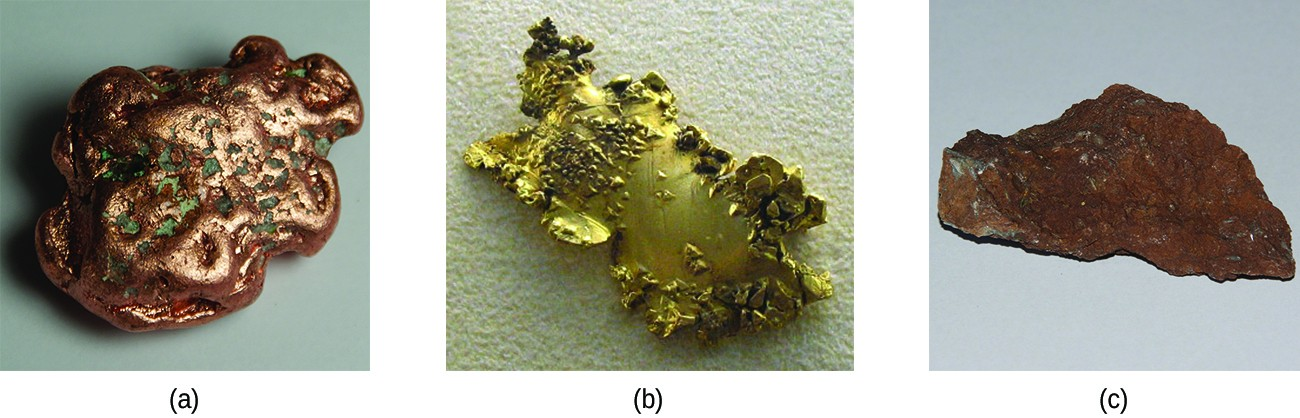Figure 4. Transition metals occur in nature in various forms. Examples include (a) a nugget of copper, (b) a deposit of gold, and (c) an ore containing oxidized iron. (credit a: modification of work by http://images-of-elements.com/copper-2.jpg; credit c: modification of work by http://images-of-elements.com/iron-ore.jpg)

Generally, the transition elements are extracted from minerals found in a variety of ores. However, the ease of their recovery varies widely, depending on the concentration of the element in the ore, the identity of the other elements present, and the difficulty of reducing the element to the free metal.

In general, it is not difficult to reduce ions of the d-block elements to the free element. Carbon is a sufficiently strong reducing agent in most cases. However, like the ions of the more active main group metals, ions of the f-block elements must be isolated by electrolysis or by reduction with an active metal such as calcium.

We shall discuss the processes used for the isolation of iron, copper, and silver because these three processes illustrate the principal means of isolating most of the d-block metals. In general, each of these processes involves three principal steps: preliminary treatment, smelting, and refining.

1. Preliminary treatment. In general, there is an initial treatment of the ores to make them suitable for the extraction of the metals. This usually involves crushing or grinding the ore, concentrating the metal-bearing components, and sometimes treating these substances chemically to convert them into compounds that are easier to reduce to the metal.
2. Smelting. The next step is the extraction of the metal in the molten state, a process called smelting, which includes reduction of the metallic compound to the metal. Impurities may be removed by the addition of a compound that forms a slag—a substance with a low melting point that can be readily separated from the molten metal.
3. Refining. The final step in the recovery of a metal is refining the metal. Low boiling metals such as zinc and mercury can be refined by distillation. When fused on an inclined table, low melting metals like tin flow away from higher-melting impurities. Electrolysis is another common method for refining metals.

## Isolation of Iron

The early application of iron to the manufacture of tools and weapons was possible because of the wide distribution of iron ores and the ease with which iron compounds in the ores could be reduced by carbon. For a long time, charcoal was the form of carbon used in the reduction process. The production and use of iron became much more widespread about 1620, when coke was introduced as the reducing agent. Coke is a form of carbon formed by heating coal in the absence of air to remove impurities.

The first step in the metallurgy of iron is usually roasting the ore (heating the ore in air) to remove water, decomposing carbonates into oxides, and converting sulfides into oxides. The oxides are then reduced in a blast furnace that is 80–100 feet high and about 25 feet in diameter in which the roasted ore, coke, and limestone (impure CaCO3) are introduced continuously into the top. Molten iron and slag are withdrawn at the bottom. The entire stock in a furnace may weigh several hundred tons.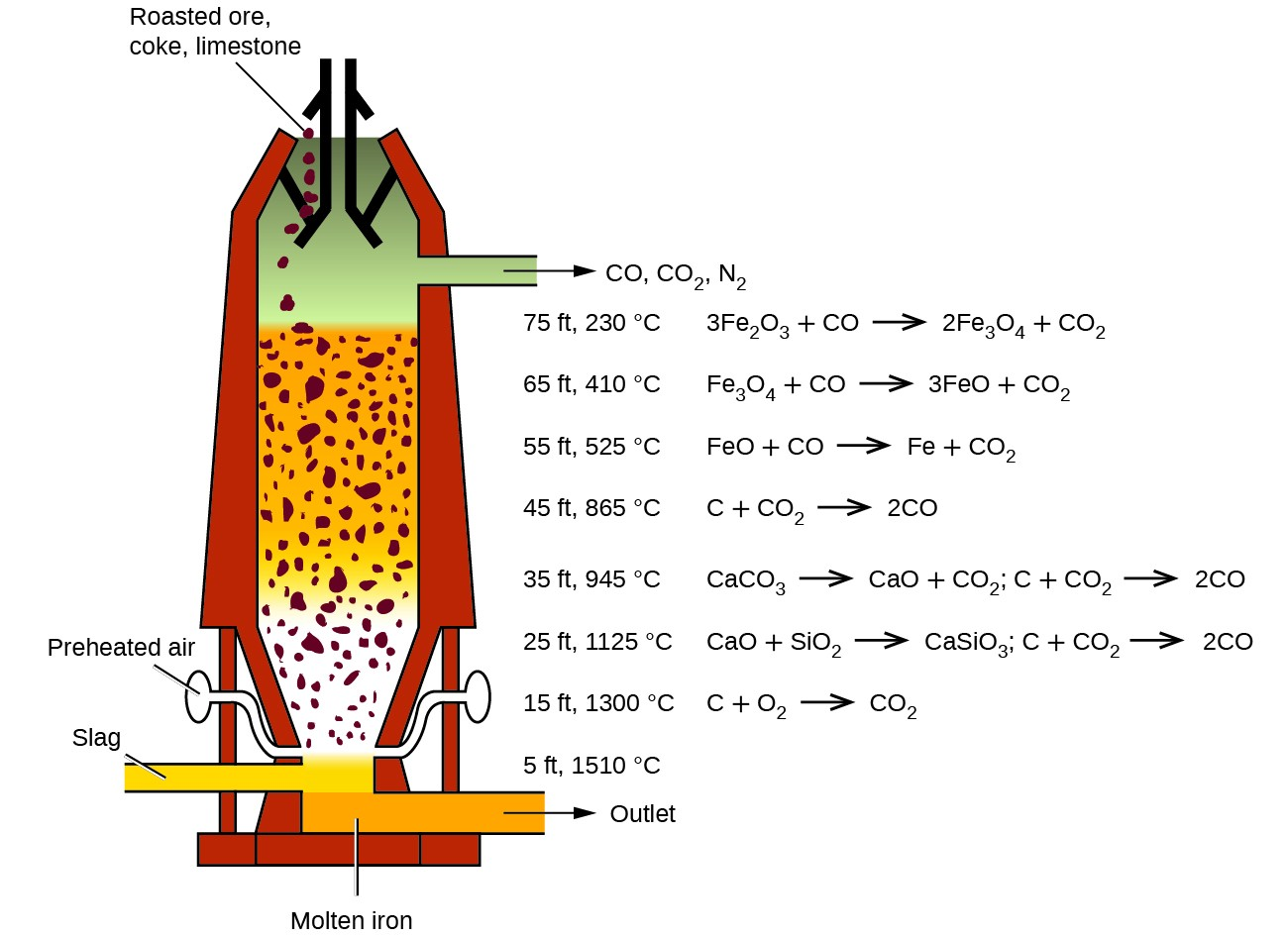Figure 5. Within a blast furnace, different reactions occur in different temperature zones. Carbon monoxide is generated in the hotter bottom regions and rises upward to reduce the iron oxides to pure iron through a series of reactions that take place in the upper regions.

Near the bottom of a furnace are nozzles through which preheated air is blown into the furnace. As soon as the air enters, the coke in the region of the nozzles is oxidized to carbon dioxide with the liberation of a great deal of heat. The hot carbon dioxide passes upward through the overlying layer of white-hot coke, where it is reduced to carbon monoxide:

${\text{CO}}_{2}\left(g\right)+\text{C}\left(s\right)\rightarrow\text{2CO}\left(g\right)$

The carbon monoxide serves as the reducing agent in the upper regions of the furnace. The individual reactions are indicated in Figure 5.

The iron oxides are reduced in the upper region of the furnace. In the middle region, limestone (calcium carbonate) decomposes, and the resulting calcium oxide combines with silica and silicates in the ore to form slag. The slag is mostly calcium silicate and contains most of the commercially unimportant components of the ore:

$\text{CaO}\left(s\right)+{\text{SiO}}_{2}\left(s\right)\rightarrow{\text{CaSiO}}_{3}\left(l\right)$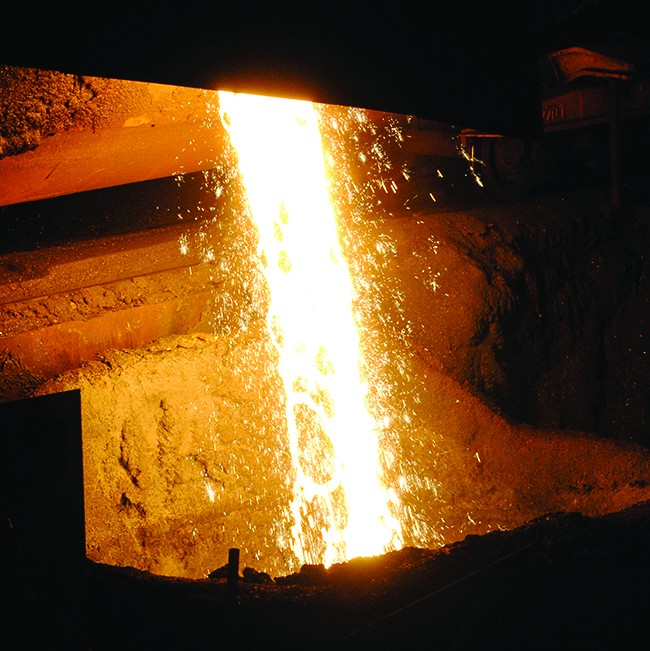Figure 6. Molten iron is shown being cast as steel. (credit: Clint Budd)

Just below the middle of the furnace, the temperature is high enough to melt both the iron and the slag. They collect in layers at the bottom of the furnace; the less dense slag floats on the iron and protects it from oxidation. Several times a day, the slag and molten iron are withdrawn from the furnace. The iron is transferred to casting machines or to a steelmaking plant.

Much of the iron produced is refined and converted into steel. Steel is made from iron by removing impurities and adding substances such as manganese, chromium, nickel, tungsten, molybdenum, and vanadium to produce alloys with properties that make the material suitable for specific uses. Most steels also contain small but definite percentages of carbon (0.04%–2.5%). However, a large part of the carbon contained in iron must be removed in the manufacture of steel; otherwise, the excess carbon would make the iron brittle.

Watch an animation of steelmaking that walks you through the process.

## Isolation of Copper

The most important ores of copper contain copper sulfides (such as covellite, CuS), although copper oxides (such as tenorite, CuO) and copper hydroxycarbonates [such as malachite, Cu2(OH)2CO3] are sometimes found. In the production of copper metal, the concentrated sulfide ore is roasted to remove part of the sulfur as sulfur dioxide. The remaining mixture, which consists of Cu2S, FeS, FeO, and SiO2, is mixed with limestone, which serves as a flux (a material that aids in the removal of impurities), and heated. Molten slag forms as the iron and silica are removed by Lewis acid-base reactions:

${\text{CaCO}}_{3}\left(s\right)+{\text{SiO}}_{2}\left(s\right)\rightarrow{\text{CaSiO}}_{3}\left(l\right)+{\text{CO}}_{2}\left(g\right)$
$\text{FeO}\left(s\right)+{\text{SiO}}_{2}\left(s\right)\rightarrow{\text{FeSiO}}_{3}\left(l\right)$

In these reactions, the silicon dioxide behaves as a Lewis acid, which accepts a pair of electrons from the Lewis base (the oxide ion).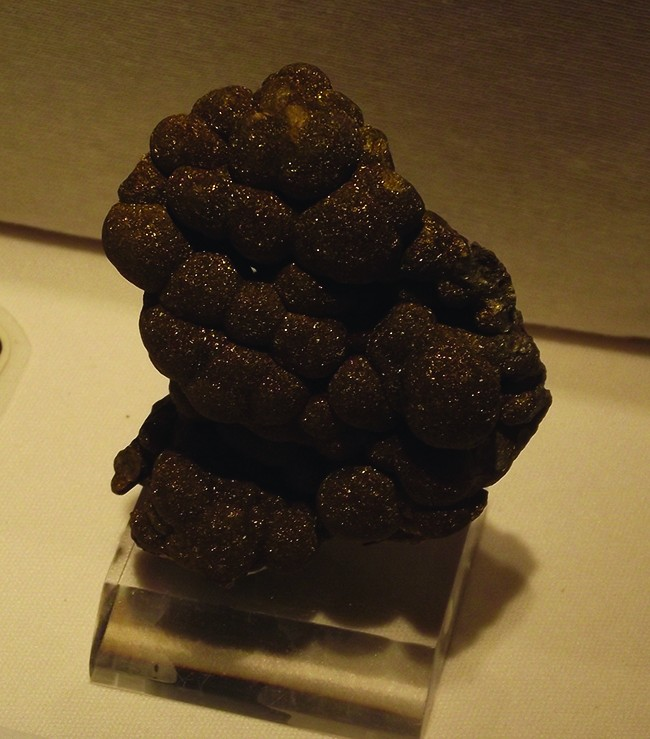Figure 7. Blister copper is obtained during the conversion of copper-containing ore into pure copper. (credit: “Tortie tude”/Wikimedia Commons)

Reduction of the Cu2S that remains after smelting is accomplished by blowing air through the molten material. The air converts part of the Cu2S into Cu2O. As soon as copper(I) oxide is formed, it is reduced by the remaining copper(I) sulfide to metallic copper:

${\text{2Cu}}_{2}\text{S}\left(l\right)+{\text{3O}}_{2}\left(g\right)\rightarrow{\text{2Cu}}_{2}\text{O}\left(l\right)+{\text{2SO}}_{2}\left(g\right)$
${\text{2Cu}}_{2}\text{O}\left(l\right)+{\text{Cu}}_{2}\text{S}\left(l\right)\rightarrow\text{6Cu}\left(l\right)+{\text{SO}}_{2}\left(g\right)$

The copper obtained in this way is called blister copper because of its characteristic appearance, which is due to the air blisters it contains. This impure copper is cast into large plates, which are used as anodes in the electrolytic refining of the metal (which is described in the chapter on electrochemistry).

## Isolation of Silver

Silver sometimes occurs in large nuggets but more frequently in veins and related deposits. At one time, panning was an effective method of isolating both silver and gold nuggets. Due to their low reactivity, these metals, and a few others, occur in deposits as nuggets. The discovery of platinum was due to Spanish explorers in Central America mistaking platinum nuggets for silver. When the metal is not in the form of nuggets, it often useful to employ a process called hydrometallurgy to separate silver from its ores. Hydrology involves the separation of a metal from a mixture by first converting it into soluble ions and then extracting and reducing them to precipitate the pure metal. In the presence of air, alkali metal cyanides readily form the soluble dicyanoargentate(I) ion, ${\left[\text{Ag}{\left(\text{CN}\right)}_{2}\right]}^{-},$ from silver metal or silver-containing compounds such as Ag2S and AgCl. Representative equations are:

$\text{4Ag}\left(s\right)+{\text{8CN}}^{-}\left(aq\right)+{\text{O}}_{2}\left(g\right)+{\text{2H}}_{2}\text{O}\left(l\right)\rightarrow 4{\left[\text{Ag}{\left(\text{CN}\right)}_{2}\right]}^{-}\left(aq\right)+{\text{4OH}}^{-}\left(aq\right)$
${\text{2Ag}}_{2}\text{S}\left(s\right)+{\text{8CN}}^{-}\left(aq\right)+{\text{O}}_{2}\left(g\right)+{\text{2H}}_{2}\text{O}\left(l\right)\rightarrow 4{\left[{\text{Ag(CN)}}_{2}\right]}^{-}\left(aq\right)+\text{2S}\left(s\right)+{\text{4OH}}^{-}\left(aq\right)$
$\text{AgCl}\left(s\right)+{\text{2CN}}^{-}\left(aq\right)\rightarrow{\left[\text{Ag}{\left(\text{CN}\right)}_{2}\right]}^{-}\left(aq\right)+{\text{Cl}}^{-}\left(aq\right)$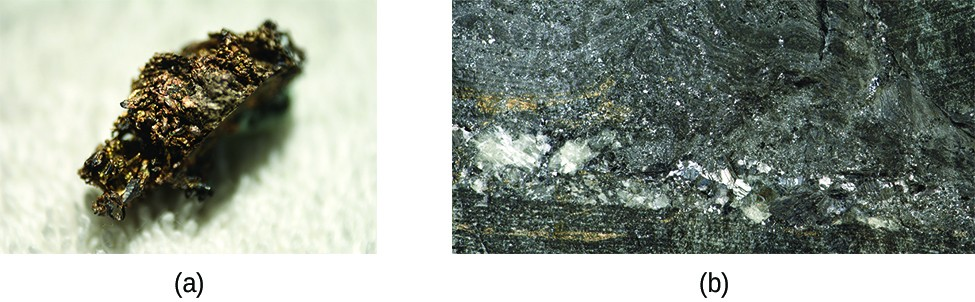Figure 8. Naturally occurring free silver may be found as nuggets (left) or in veins (right). (credit a: modification of work by “Teravolt”/Wikimedia Commons; credit b: modification of work by James St. John)

The silver is precipitated from the cyanide solution by the addition of either zinc or iron(II) ions, which serves as the reducing agent:

$2{\left[\text{Ag}{\left(\text{CN}\right)}_{2}\right]}^{-}\left(aq\right)+\text{Zn}\left(s\right)\rightarrow\text{2Ag}\left(s\right)+{\left[\text{Zn}{\left(\text{CN}\right)}_{4}\right]}^{2-}\left(aq\right)$

### Example 3: Refining Redox

One of the steps for refining silver involves converting silver into dicyanoargenate(I) ions:

$\text{4Ag}\left(s\right)+{\text{8CN}}^{-}\left(aq\right)+{\text{O}}_{2}\left(g\right)+2{\text{H}}_{2}\text{O}\left(l\right)\rightarrow 4{\left[\text{Ag}{\left(\text{CN}\right)}_{2}\right]}^{-}\left(aq\right)+{\text{4OH}}^{-}\left(aq\right)$

Explain why oxygen must be present to carry out the reaction. Why does the reaction not occur as:

$\text{4Ag}\left(s\right)+{\text{8CN}}^{-}\left(aq\right)\rightarrow 4{\left[\text{Ag}{\left(\text{CN}\right)}_{2}\right]}^{-}\left(aq\right)\text{?}$

During the refining of iron, carbon must be present in the blast furnace. Why is carbon necessary to convert iron oxide into iron?

## Transition Metal Compounds

The bonding in the simple compounds of the transition elements ranges from ionic to covalent. In their lower oxidation states, the transition elements form ionic compounds; in their higher oxidation states, they form covalent compounds or polyatomic ions. The variation in oxidation states exhibited by the transition elements gives these compounds a metal-based, oxidation-reduction chemistry. The chemistry of several classes of compounds containing elements of the transition series follows.

### Halides

Anhydrous halides of each of the transition elements can be prepared by the direct reaction of the metal with halogens. For example:

$\text{2Fe}\left(s\right)+{\text{3Cl}}_{2}\left(g\right)\rightarrow{\text{2FeCl}}_{3}\left(s\right)$

Heating a metal halide with additional metal can be used to form a halide of the metal with a lower oxidation state:

$\text{Fe}\left(s\right)+{\text{2FeCl}}_{3}\left(s\right)\rightarrow{\text{3FeCl}}_{2}\left(s\right)$

The stoichiometry of the metal halide that results from the reaction of the metal with a halogen is determined by the relative amounts of metal and halogen and by the strength of the halogen as an oxidizing agent. Generally, fluorine forms fluoride-containing metals in their highest oxidation states. The other halogens may not form analogous compounds.

In general, the preparation of stable water solutions of the halides of the metals of the first transition series is by the addition of a hydrohalic acid to carbonates, hydroxides, oxides, or other compounds that contain basic anions. Sample reactions are:

${\text{NiCO}}_{3}\left(s\right)+\text{2HF}\left(aq\right)\rightarrow{\text{NiF}}_{2}\left(aq\right)+{\text{H}}_{2}\text{O}\left(l\right)+{\text{CO}}_{2}\left(g\right)$
$\text{Co}{\left(\text{OH}\right)}_{2}\left(s\right)+\text{2 HBr}\left(aq\right)\rightarrow{\text{CoBr}}_{2}\left(aq\right)+2{\text{H}}_{2}\text{O}\left(l\right)$

Most of the first transition series metals also dissolve in acids, forming a solution of the salt and hydrogen gas. For example:

$\text{Cr}\left(s\right)+\text{2HCl}\left(aq\right)\rightarrow{\text{CrCl}}_{2}\left(aq\right)+{\text{H}}_{2}\left(g\right)$

The polarity of bonds with transition metals varies based not only upon the electronegativities of the atoms involved but also upon the oxidation state of the transition metal. Remember that bond polarity is a continuous spectrum with electrons being shared evenly (covalent bonds) at one extreme and electrons being transferred completely (ionic bonds) at the other. No bond is ever 100% ionic, and the degree to which the electrons are evenly distributed determines many properties of the compound. Transition metal halides with low oxidation numbers form more ionic bonds. For example, titanium(II) chloride and titanium(III) chloride (TiCl2 and TiCl3) have high melting points that are characteristic of ionic compounds, but titanium(IV) chloride (TiCl4) is a volatile liquid, consistent with having covalent titanium-chlorine bonds. All halides of the heavier d-block elements have significant covalent characteristics.

The covalent behavior of the transition metals with higher oxidation states is exemplified by the reaction of the metal tetrahalides with water. Like covalent silicon tetrachloride, both the titanium and vanadium tetrahalides react with water to give solutions containing the corresponding hydrohalic acids and the metal oxides:

${\text{SiCl}}_{4}\left(l\right)+2{\text{H}}_{2}\text{O}\left(l\right)\rightarrow{\text{SiO}}_{2}\left(s\right)+\text{4HCl}\left(aq\right)$
${\text{TiCl}}_{4}\left(l\right)+2{\text{H}}_{2}\text{O}\left(l\right)\rightarrow{\text{TiO}}_{2}\left(s\right)+\text{4HCl}\left(aq\right)$

### Oxides

As with the halides, the nature of bonding in oxides of the transition elements is determined by the oxidation state of the metal. Oxides with low oxidation states tend to be more ionic, whereas those with higher oxidation states are more covalent. These variations in bonding are because the electronegativities of the elements are not fixed values. The electronegativity of an element increases with increasing oxidation state. Transition metals in low oxidation states have lower electronegativity values than oxygen; therefore, these metal oxides are ionic. Transition metals in very high oxidation states have electronegativity values close to that of oxygen, which leads to these oxides being covalent.

The oxides of the first transition series can be prepared by heating the metals in air. These oxides are Sc2O3, TiO2, V2O5, Cr2O3, Mn3O4, Fe3O4, Co3O4, NiO, and CuO.

Alternatively, these oxides and other oxides (with the metals in different oxidation states) can be produced by heating the corresponding hydroxides, carbonates, or oxalates in an inert atmosphere. Iron(II) oxide can be prepared by heating iron(II) oxalate, and cobalt(II) oxide is produced by heating cobalt(II) hydroxide:

${\text{FeC}}_{2}{\text{O}}_{4}\left(s\right)\rightarrow\text{FeO}\left(s\right)+\text{CO}\left(g\right)+{\text{CO}}_{2}\left(g\right)$
${\text{Co(OH)}}_{2}\left(s\right)\rightarrow\text{CoO}\left(s\right)+{\text{H}}_{2}\text{O}\left(g\right)$

With the exception of CrO3 and Mn2O7, transition metal oxides are not soluble in water. They can react with acids and, in a few cases, with bases. Overall, oxides of transition metals with the lowest oxidation states are basic (and react with acids), the intermediate ones are amphoteric, and the highest oxidation states are primarily acidic. Basic metal oxides at a low oxidation state react with aqueous acids to form solutions of salts and water. Examples include the reaction of cobalt(II) oxide accepting protons from nitric acid, and scandium(III) oxide accepting protons from hydrochloric acid:

$\text{CoO}\left(s\right)+{\text{2HNO}}_{3}\left(aq\right)\rightarrow\text{Co}{\left({\text{NO}}_{3}\right)}_{2}\left(aq\right)+{\text{H}}_{2}\text{O}\left(l\right)$
${\text{Sc}}_{2}{\text{O}}_{3}\left(s\right)+\text{6HCl}\left(aq\right)\rightarrow{\text{2ScCl}}_{3}\left(aq\right)+{\text{3H}}_{2}\text{O}\left(l\right)$

The oxides of metals with oxidation states of 4+ are amphoteric, and most are not soluble in either acids or bases. Vanadium(V) oxide, chromium(VI) oxide, and manganese(VII) oxide are acidic. They react with solutions of hydroxides to form salts of the oxyanions ${\text{VO}}_{4}{}^{3-},$ ${\text{CrO}}_{4}{}^{2-},$ and ${\text{MnO}}_{4}{}^{-}.$ For example, the complete ionic equation for the reaction of chromium(VI) oxide with a strong base is given by:

${\text{CrO}}_{3}\left(s\right)+{\text{2Na}}^{+}\left(aq\right)+{\text{2OH}}^{-}\left(aq\right)\rightarrow{\text{2Na}}^{+}\left(aq\right)+{\text{CrO}}_{4}{}^{2-}\left(aq\right)+{\text{H}}_{2}\text{O}\left(l\right)$

Chromium(VI) oxide and manganese(VII) oxide react with water to form the acids H2CrO4 and HMnO4, respectively.

### Hydroxides

When a soluble hydroxide is added to an aqueous solution of a salt of a transition metal of the first transition series, a gelatinous precipitate forms. For example, adding a solution of sodium hydroxide to a solution of cobalt sulfate produces a gelatinous pink or blue precipitate of cobalt(II) hydroxide. The net ionic equation is:

${\text{Co}}^{2+}\left(aq\right)+{\text{2OH}}^{-}\left(aq\right)\rightarrow\text{Co}{\left(\text{OH}\right)}_{2}\left(s\right)$

In this and many other cases, these precipitates are hydroxides containing the transition metal ion, hydroxide ions, and water coordinated to the transition metal. In other cases, the precipitates are hydrated oxides composed of the metal ion, oxide ions, and water of hydration:

${\text{4Fe}}^{3+}\left(aq\right)+{\text{6OH}}^{-}\left(aq\right)+\text{n}{\text{H}}_{2}\text{O}\left(l\right)\rightarrow 2{\text{Fe}}_{2}{\text{O}}_{3}\cdot\left(\text{n}+3\right){\text{H}}_{2}\text{O}\left(s\right)$

These substances do not contain hydroxide ions. However, both the hydroxides and the hydrated oxides react with acids to form salts and water. When precipitating a metal from solution, it is necessary to avoid an excess of hydroxide ion, as this may lead to complex ion formation as discussed later in this chapter. The precipitated metal hydroxides can be separated for further processing or for waste disposal.

### Carbonates

Many of the elements of the first transition series form insoluble carbonates. It is possible to prepare these carbonates by the addition of a soluble carbonate salt to a solution of a transition metal salt. For example, nickel carbonate can be prepared from solutions of nickel nitrate and sodium carbonate according to the following net ionic equation:

${\text{Ni}}^{2+}\left(aq\right)+{\text{CO}}_{3}{}^{2-}\rightarrow{\text{NiCO}}_{3}\left(s\right)$

The reactions of the transition metal carbonates are similar to those of the active metal carbonates. They react with acids to form metals salts, carbon dioxide, and water. Upon heating, they decompose, forming the transition metal oxides.

### Other Salts

In many respects, the chemical behavior of the elements of the first transition series is very similar to that of the main group metals. In particular, the same types of reactions that are used to prepare salts of the main group metals can be used to prepare simple ionic salts of these elements.

A variety of salts can be prepared from metals that are more active than hydrogen by reaction with the corresponding acids: Scandium metal reacts with hydrobromic acid to form a solution of scandium bromide:

$\text{2Sc}\left(s\right)+\text{6HBr}\left(aq\right)\rightarrow 2{\text{ScBr}}_{3}\left(aq\right)+{\text{3H}}_{2}\left(g\right)$

The common compounds that we have just discussed can also be used to prepare salts. The reactions involved include the reactions of oxides, hydroxides, or carbonates with acids. For example:

$\text{Ni}{\left(\text{OH}\right)}_{2}\left(s\right)+2{\text{H}}_{3}{\text{O}}^{+}\left(aq\right)+{\text{2ClO}}_{4}{}^{-}\left(aq\right)\rightarrow{\text{Ni}}^{2+}\left(aq\right)+{\text{2ClO}}_{4}{}^{-}\left(aq\right)+{\text{4H}}_{2}\text{O}\left(l\right)$

Substitution reactions involving soluble salts may be used to prepare insoluble salts. For example:

${\text{Ba}}^{2+}\left(aq\right)+{\text{2Cl}}^{-}\left(aq\right)+{\text{2K}}^{+}\left(aq\right)+{\text{CrO}}_{4}{}^{2-}\left(aq\right)\rightarrow{\text{BaCrO}}_{4}\left(s\right)+{\text{2K}}^{+}\left(aq\right)+{\text{2Cl}}^{-}\left(aq\right)$

In our discussion of oxides in this section, we have seen that reactions of the covalent oxides of the transition elements with hydroxides form salts that contain oxyanions of the transition elements.

### High Temperature Superconductors

A superconductor is a substance that conducts electricity with no resistance. This lack of resistance means that there is no energy loss during the transmission of electricity. This would lead to a significant reduction in the cost of electricity.

Most currently used, commercial superconducting materials, such as NbTi and Nb3Sn, do not become superconducting until they are cooled below 23 K (−250 °C). This requires the use of liquid helium, which has a boiling temperature of 4 K and is expensive and difficult to handle. The cost of liquid helium has deterred the widespread application of superconductors.

One of the most exciting scientific discoveries of the 1980s was the characterization of compounds that exhibit superconductivity at temperatures above 90 K. (Compared to liquid helium, 90 K is a high temperature.) Typical among the high-temperature superconducting materials are oxides containing yttrium (or one of several rare earth elements), barium, and copper in a 1:2:3 ratio. The formula of the ionic yttrium compound is YBa2Cu3O7.

The new materials become superconducting at temperatures close to 90 K, temperatures that can be reached by cooling with liquid nitrogen (boiling temperature of 77 K). Not only are liquid nitrogen-cooled materials easier to handle, but the cooling costs are also about 1000 times lower than for liquid helium.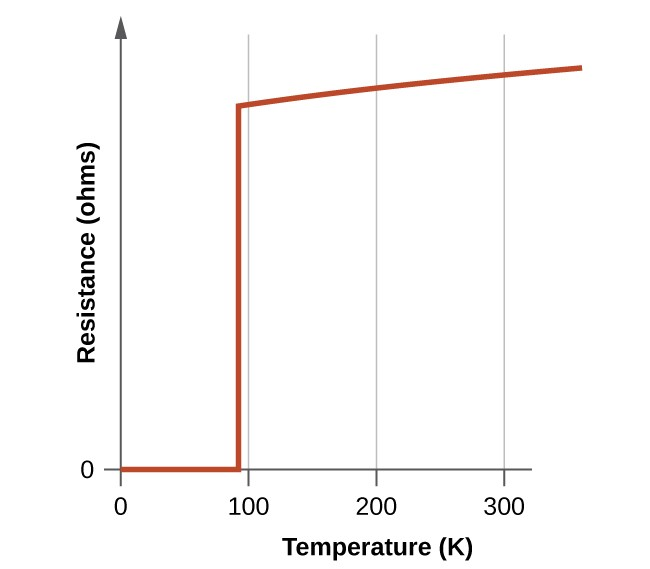Figure 9. The resistance of the high-temperature superconductor YBa2Cu3O7 varies with temperature. Note how the resistance falls to zero below 92 K, when the substance becomes superconducting.

Although the brittle, fragile nature of these materials presently hampers their commercial applications, they have tremendous potential that researchers are hard at work improving their processes to help realize. Superconducting transmission lines would carry current for hundreds of miles with no loss of power due to resistance in the wires. This could allow generating stations to be located in areas remote from population centers and near the natural resources necessary for power production. The first project demonstrating the viability of high-temperature superconductor power transmission was established in New York in 2008.

Researchers are also working on using this technology to develop other applications, such as smaller and more powerful microchips. In addition, high-temperature superconductors can be used to generate magnetic fields for applications such as medical devices, magnetic levitation trains, and containment fields for nuclear fusion reactors.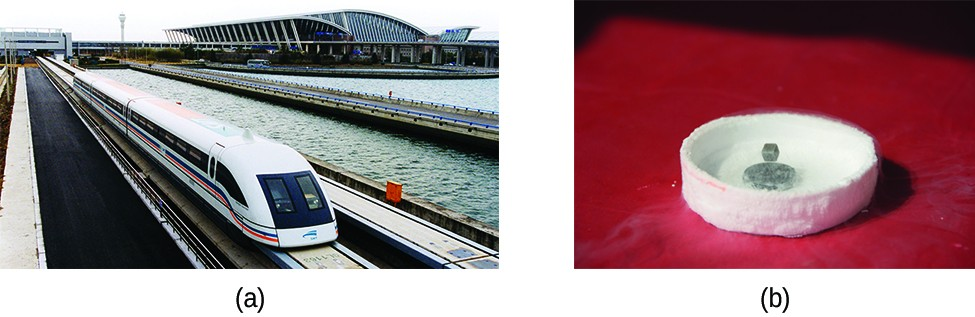Figure 10. This magnetic levitation train (or maglev) uses superconductor technology to move along its tracks. (credit a: modification of work by Alex Needham; credit b: modification of work by Kevin Jarrett)

Watch how a high-temperature superconductor levitates around a magnetic racetrack in the video.

### Key Concepts and Summary

The transition metals are elements with partially filled d orbitals, located in the d-block of the periodic table. The reactivity of the transition elements varies widely from very active metals such as scandium and iron to almost inert elements, such as the platinum metals. The type of chemistry used in the isolation of the elements from their ores depends upon the concentration of the element in its ore and the difficulty of reducing ions of the elements to the metals. Metals that are more active are more difficult to reduce.

Transition metals exhibit chemical behavior typical of metals. For example, they oxidize in air upon heating and react with elemental halogens to form halides. Those elements that lie above hydrogen in the activity series react with acids, producing salts and hydrogen gas. Oxides, hydroxides, and carbonates of transition metal compounds in low oxidation states are basic. Halides and other salts are generally stable in water, although oxygen must be excluded in some cases. Most transition metals form a variety of stable oxidation states, allowing them to demonstrate a wide range of chemical reactivity.

### Exercises

1. Write the electron configurations for each of the following elements:
1. Sc
2. Ti
3. Cr
4. Fe
5. Ru
2. Write the electron configurations for each of the following elements and its ions:
1. Ti
2. Ti2+
3. Ti3+
4. Ti4+
3. Write the electron configurations for each of the following elements and its 3+ ions:
1. La
2. Sm
3. Lu
4. Why are the lanthanoid elements not found in nature in their elemental forms?
5. Which of the following elements is most likely to be used to prepare La by the reduction of La2O3: Al, C, or Fe? Why?
6. Which of the following is the strongest oxidizing agent: ${\text{VO}}_{4}{}^{3},$ ${\text{CrO}}_{4}{}^{2-}$, or ${\text{MnO}}_{4}{}^{-}$?
7. Which of the following elements is most likely to form an oxide with the formula MO3: Zr, Nb, or Mo?
8. The following reactions all occur in a blast furnace. Which of these are redox reactions?
1. ${\text{3Fe}}_{2}{\text{O}}_{3}\left(s\right)+\text{CO}\left(g\right)\rightarrow{\text{2Fe}}_{3}{\text{O}}_{4}\left(s\right)+{\text{CO}}_{2}\left(g\right)$
2. ${\text{Fe}}_{3}{\text{O}}_{4}\left(s\right)+\text{CO}\left(g\right)\rightarrow\text{3FeO}\left(s\right)+{\text{CO}}_{2}\left(g\right)$
3. $\text{FeO}\left(s\right)+\text{CO}\left(g\right)\rightarrow\text{Fe}\left(l\right)+{\text{CO}}_{2}\left(g\right)$
4. $\text{C}\left(s\right)+{\text{O}}_{2}\left(g\right)\rightarrow{\text{CO}}_{2}\left(g\right)$
5. $\text{C}\left(s\right)+{\text{CO}}_{2}\left(g\right)\rightarrow\text{2CO}\left(g\right)$
6. ${\text{CaCO}}_{3}\left(s\right)\rightarrow\text{CaO}\left(s\right)+{\text{CO}}_{2}\left(g\right)$
7. $\text{CaO}\left(s\right)+{\text{SiO}}_{2}\left(s\right)\rightarrow{\text{CaSiO}}_{3}\left(l\right)$
9. Why is the formation of slag useful during the smelting of iron?
10. Would you expect an aqueous manganese(VII) oxide solution to have a pH greater or less than 7.0? Justify your answer.
11. Iron(II) can be oxidized to iron(III) by dichromate ion, which is reduced to chromium(III) in acid solution. A 2.5000-g sample of iron ore is dissolved and the iron converted into iron(II). Exactly 19.17 mL of 0.0100 M Na2Cr2O7 is required in the titration. What percentage of the ore sample was iron?
12. How many cubic feet of air at a pressure of 760 torr and 0 °C is required per ton of Fe2O3 to convert that Fe2O3 into iron in a blast furnace? For this exercise, assume air is 19% oxygen by volume.
13. Find the potentials of the following electrochemical cell: Cd | Cd2+, $\text{M}=0.10$ ‖ Ni2+, $\text{M}=0.50$ | Ni
14. The standard reduction potential for the reaction ${\left[{\text{Co(H}}_{2}\text{O)}_{6}\right]}^{3+}\left(aq\right)+{\text{e}}^{-}\rightarrow{\left[{\text{Co(H}}_{2}\text{O)}_{6}\right]}^{2+}\left(aq\right)$ is about 1.8 V. The reduction potential for the reaction ${\left[{\text{Co(NH}}_{3}\text{)}_{6}\right]}^{3+}\left(aq\right)+{\text{e}}^{-}\rightarrow{\left[{\text{Co(NH}}_{3}\text{)}_{6}\right]}^{2+}\left(aq\right)$ is +0.1 V. Calculate the cell potentials to show whether the complex ions, ${\left[\text{Co}{\left({\text{H}}_{2}\text{O}\right)}_{6}\right]}^{2+}$ and/or ${\left[\text{Co}{\left({\text{NH}}_{3}\right)}_{6}\right]}^{2+},$ can be oxidized to the corresponding cobalt(III) complex by oxygen.
15. Predict the products of each of the following reactions. (Note: In addition to using the information in this chapter, also use the knowledge you have accumulated at this stage of your study, including information on the prediction of reaction products.)
1. ${\text{MnCO}}_{3}\left(s\right)+\text{HI}\left(aq\right)\rightarrow$
2. $\text{CoO}\left(s\right)+{\text{O}}_{2}\left(g\right)\rightarrow$
3. $\text{La}\left(s\right)+{\text{O}}_{2}\left(g\right)\rightarrow$
4. $\text{V}\left(s\right)+{\text{VCl}}_{4}\left(s\right)\rightarrow$
5. $\text{Co}\left(s\right)+{\text{xsF}}_{2}\left(g\right)\rightarrow$
6. ${\text{CrO}}_{3}\left(s\right)+\text{CsOH}\left(aq\right)\rightarrow$
16. Predict the products of each of the following reactions. (Note: In addition to using the information in this chapter, also use the knowledge you have accumulated at this stage of your study, including information on the prediction of reaction products.)
1. $\text{Fe}\left(s\right)+{\text{H}}_{2}{\text{SO}}_{4}\left(aq\right)\rightarrow$
2. ${\text{FeCl}}_{3}\left(aq\right)+\text{NaOH}\left(aq\right)\rightarrow$
3. $\text{Mn}{\left(\text{OH}\right)}_{2}\left(s\right)+\text{HBr}\left(aq\right)\rightarrow$
4. $\text{Cr}\left(s\right)+{\text{O}}_{2}\left(g\right)\rightarrow$
5. ${\text{Mn}}_{2}{\text{O}}_{3}\left(s\right)+\text{HCl}\left(aq\right)\rightarrow$
6. $\text{Ti}\left(s\right)+xs{\text{F}}_{2}\left(g\right)\rightarrow$
17. Describe the electrolytic process for refining copper.
18. Predict the products of the following reactions and balance the equations.
1. Zn is added to a solution of Cr2(SO4)3 in acid.
2. FeCl2 is added to a solution containing an excess of ${\text{Cr}}_{2}{\text{O}}_{7}{}^{2-}$ in hydrochloric acid.
3. Cr2+ is added to ${\text{Cr}}_{2}{\text{O}}_{7}{}^{2-}$ in acid solution.
4. Mn is heated with CrO3.
5. CrO is added to 2HNO3 in water.
6. FeCl3 is added to an aqueous solution of NaOH.
19. What is the gas produced when iron(II) sulfide is treated with a nonoxidizing acid?
20. Predict the products of each of the following reactions and then balance the chemical equations.
1. Fe is heated in an atmosphere of steam.
2. NaOH is added to a solution of Fe(NO3)3.
3. FeSO4 is added to an acidic solution of KMnO4.
4. Fe is added to a dilute solution of H2SO4.
5. A solution of Fe(NO3)2 and HNO3 is allowed to stand in air.
6. FeCO3 is added to a solution of HClO4.
7. Fe is heated in air.
21. Balance the following equations by oxidation-reduction methods; note that three elements change oxidation state.
$\text{Co}{\left({\text{NO}}_{3}\right)}_{2}\left(s\right)\rightarrow{\text{Co}}_{2}{\text{O}}_{3}\left(s\right)+{\text{NO}}_{2}\left(g\right)+{\text{O}}_{2}\left(g\right)$
22. Dilute sodium cyanide solution is slowly dripped into a slowly stirred silver nitrate solution. A white precipitate forms temporarily but dissolves as the addition of sodium cyanide continues. Use chemical equations to explain this observation. Silver cyanide is similar to silver chloride in its solubility.
23. Predict which will be more stable, ${\left[{\text{CrO}}_{4}\right]}^{2-}$ or ${\left[{\text{WO}}_{4}\right]}^{2-},$ and explain.
24. Give the oxidation state of the metal for each of the following oxides of the first transition series. (Hint: Oxides of formula M3O4 are examples of mixed valence compounds in which the metal ion is present in more than one oxidation state. It is possible to write these compound formulas in the equivalent format MO•M2O3, to permit estimation of the metal’s two oxidation states.)
1. Sc2O3
2. TiO2
3. V2O5
4. CrO3
5. MnO2
6. Fe3O4
7. Co3O4
8. NiO
9. Cu2O

## Glossary

actinide series (also, actinoid series): actinium and the elements in the second row or the f-block, atomic numbers 89–103

coordination compound: stable compound in which the central metal atom or ion acts as a Lewis acid and accepts one or more pairs of electrons

d-block element: one of the elements in groups 3–11 with valence electrons in d orbitals

f-block element: (also, inner transition element) one of the elements with atomic numbers 58–71 or 90–103 that have valence electrons in f orbitals; they are frequently shown offset below the periodic table

first transition series: transition elements in the fourth period of the periodic table (first row of the d-block), atomic numbers 21–29

fourth transition series: transition elements in the seventh period of the periodic table (fourth row of the d-block), atomic numbers 89 and 104–111

hydrometallurgy: process in which a metal is separated from a mixture by first converting it into soluble ions, extracting the ions, and then reducing the ions to precipitate the pure metal

lanthanide series (also, lanthanoid series): lanthanum and the elements in the first row or the f-block, atomic numbers 57–71

platinum metals: group of six transition metals consisting of ruthenium, osmium, rhodium, iridium, palladium, and platinum that tend to occur in the same minerals and demonstrate similar chemical properties

rare earth element: collection of 17 elements including the lanthanides, scandium, and yttrium that often occur together and have similar chemical properties, making separation difficult

second transition series: transition elements in the fifth period of the periodic table (second row of the d-block), atomic numbers 39–47

smelting: process of extracting a pure metal from a molten ore

steel: material made from iron by removing impurities in the iron and adding substances that produce alloys with properties suitable for specific uses

superconductor: material that conducts electricity with no resistance

third transition series: transition elements in the sixth period of the periodic table (third row of the d-block), atomic numbers 57 and 72–79